# 工程數學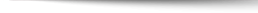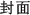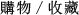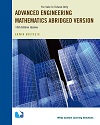## Advanced Engineering Mathematics Abridged Version 10/e Update (Taiwan Custom Version)

+作者：

### Kreyszig

+年份：
2018 年10 版
+ISBN：
9781119934172
+書號：
MA0459PCTS
+規格：

+頁數：
924
+出版商：
John Wiley

\$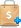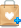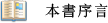●Revised Problem Sets: This edition includes an extensive revision of the problem sets, making them even more effective, useful, and up-to-date.
Chapter Introductions: These have also been rewritten to be more accessible and helpful to students.
Rewrites: Some material has been rewritten specifically to better help students draw conclusions and tackle more advanced material.
Chapter Revisions: Many of the chapters in this edition have been rewritten entirely.  Some have had material added, including but not limited to:
Introduction of Euler’s Method in section 1.2
Partial Derivatives on a Surface in section 9.6
Introduction to the Heat Equation in section 12.5●Simplicity of Examples: To make the book teachable, why choose complicated examples when well-written simple ones are as instructive or even better?
Independence of Chapters: To provide flexibility in tailoring courses to special needs.
Self-Contained Presentation: Except for a few clearly marked places where a proof would exceed the level of the book and a reference is given instead.
Modern Standard Notation: To help students with other courses, modern books, and mathematical and engineering journals.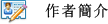Erwin Kreyszig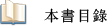PART A Ordinary Differential Equations (ODEs)
CHAPTER 1 First-Order ODEs
CHAPTER 2 Second-Order Linear ODEs
CHAPTER 3 Higher Order Linear ODEs
CHAPTER 4 Systems of ODEs. Phase Plane. Qualitative Methods
CHAPTER 5 Series Solutions of ODEs. Special Functions
CHAPTER 6 Laplace Transforms
PART B Linear Algebra. Vector Calculus
CHAPTER 7 Linear Algebra: Matrices, Vectors, Determinants. Linear Systems
CHAPTER 8 Linear Algebra: Matrix Eigenvalue Problems
CHAPTER 9 Vector Differential Calculus. Grad, Div, Curl
CHAPTER 10 Vector Integral Calculus. Integral Theorems
PART C Fourier Analysis. Partial Differential Equations (PDEs)
CHAPTER 11 Fourier Analysis
CHAPTER 12 Partial Differential Equations (PDEs)
PART D Complex Analysis
CHAPTER 13 Complex Numbers and Functions. Complex Differentiaion
CHAPTER 14 Complex Integration
CHAPTER 15 Power Series, Taylor Series
CHAPTER 16 Laurent Series. Residue Integration
CHAPTER 17 Conformal Mapping
CHAPTER 18 Complex Analysis and Potential Theory